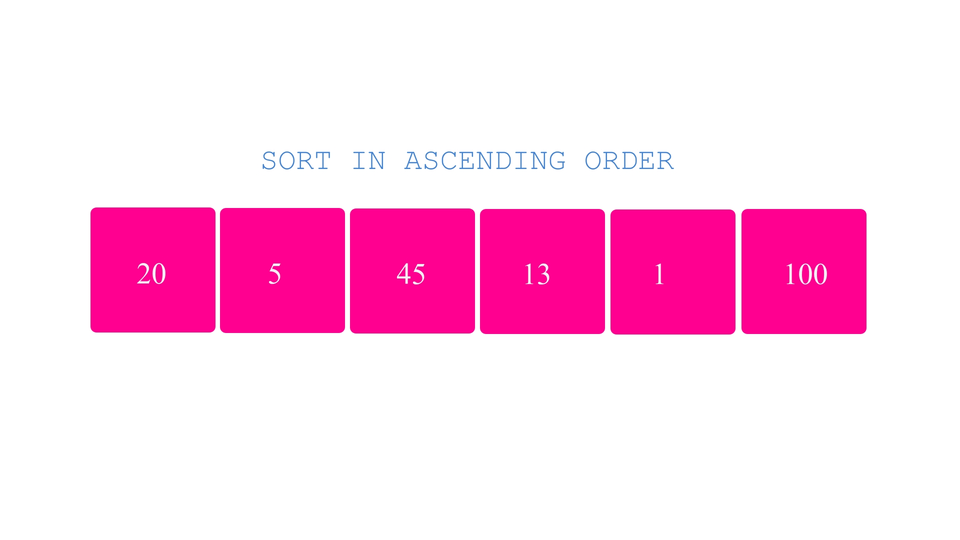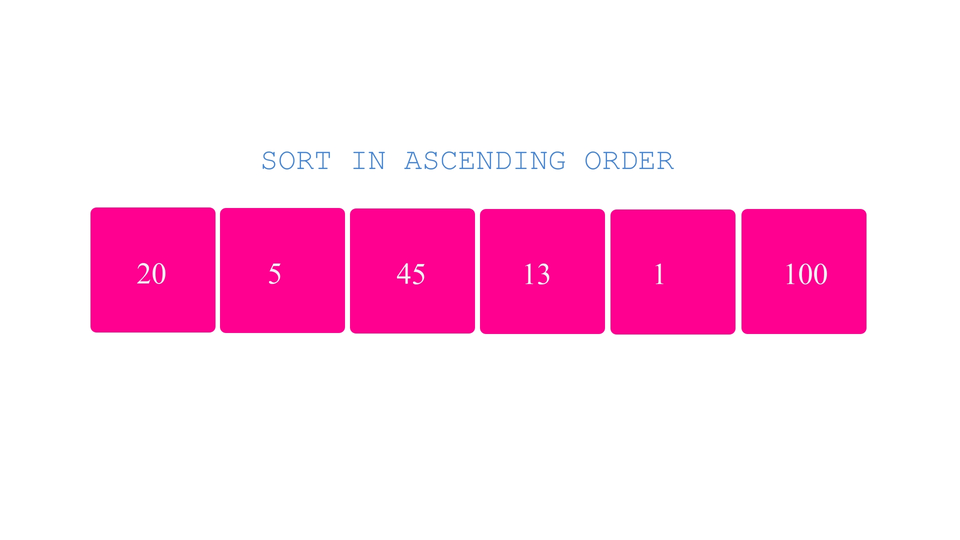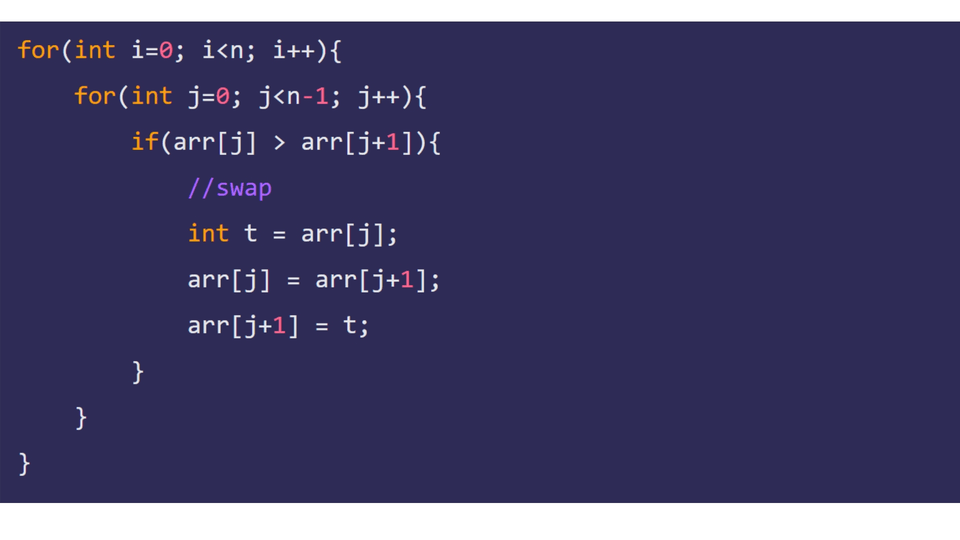# Bubble SortCreated September 3, 2021

In this article I'm gonna talk about Bubble Sort Algorithm. It is one of the simplest Sorting Algorithms there exists for sorting.

Let's get started!

## What is Bubble Sort?

Bubble sort is a sorting technique which compares and swaps(if needed) each pair of adjacent elements present in an array repeatedly until all the adjacent element pairs are arranged in the required order.

This algorithm is called Bubble Sort because the largest/smallest element is moved to the end of the array by the end of the whole process just like how a bubble floats up to the surface of water.

## Algorithm

Now suppose you've been given this puzzle which contains numbered blocks. You cant move these blocks in any way but swap them with the adjacent element. You've been given this task to arrange the numbers written on them in ascending order. How would you do it?

Probably, you will -

1. Start from the first element.
2. compare the first element with the second element, swap if not in right order.
3. then compare the second element with the third element, swap if not in right order.
4. then compare the third and fourth element, swap if not in right order.

And so on.... Until you reach the last element of the array. Then repeat all the steps again and again until all the elements are in the right order.Right?

Summing up the whole method, following are the steps that you can follow -

1. Let a variable "i" be equal to 0(first element). Let array length be "n".
2. Start from the first element(varibale j = 0).
3. Compare j'th element with j+1'th element until j reaches "n-2"(the second last index value).
4. Increase value of i by 1.
5. Repeat the steps from 2 to 4 until i == n-1(last element). Here's an explanatory illustration for the same-## The Code

Following is the code to sort a user entered array in ascending order using Bubble Sort

``````for(int i=0; i<n; i++){
for(int j=0; j<n-1; j++){
if(arr[j] > arr[j+1]){
//swap
int t = arr[j];
arr[j] = arr[j+1];
arr[j+1] = t;
}
}
}
``````

You can try implementing Bubble Sort to sort elements in Descending Order too.(Hint: Just flip the comparison symbol in the if statement)

Here's the full code, including the getting the array elements from the user and displaying the final array -

``````#include <stdio.h>

int main(){
int n;

printf("Enter number of element: ");
scanf(" %d", &n);

int arr[n];

//get the array elements from the user:
for(int i=0; i<n; i++){
printf("Enter element %d: ",i+1);
scanf(" %d", &arr[i]);
}

//display the array before sorting:
printf("Unsorted array: ");
for(int i=0; i<n; i++){
printf("%d ", arr[i]);
}printf("\n");

//to arrange in ascending order
for(int i=0; i<n; i++){
for(int j=0; j<n-1; j++){
if(arr[j] > arr[j+1]){
//swap
int t = arr[j];
arr[j] = arr[j+1];
arr[j+1] = t;
}
}
}

//display the sorted array
printf("Sorted array: ");
for(int i=0; i<n; i++){
printf("%d ", arr[i]);
}printf("\n");
}
``````

## Complexity

Time complexity for a single for loop depends on the number of times the for loop will execute. For a for loop counter variable ranging from 1 to n the time complexity is O(n).

So, in this problem, the inner for loop will execute n times for each time the outer for loop executes. That is, the complexity comes out to be O(nxn) or O(n²).The disadvantage of using this algorithm is that the loop would execute exactly the same amount of time even if the whole array is already sorted. To stop that from happening, we can add a break command if no swaps happens while executing the inner for loop.

## Conclusion

Bubble Sorting is a very simple algorithm and easy to understand. This might even be the first approach that would have come to your mind when you were introduced to the concept of sorting while learning to code. One of the disadvantages of this algorithm is that it is very slow due to O(n).